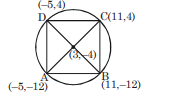# A square is inscribed inthe circle`
Question:

A square is inscribed inthe circle

$x^{2}+y^{2}-6 x+8 y-103=0$ with its sides parallel to the corrdinate axes. Then the distance of the vertex of this square which is nearest to the origin is :-

1. 13

2. $\sqrt{137}$

3. 6

4. $\sqrt{41}$

Correct Option: , 4

Solution:

$\mathrm{R}=\sqrt{9+16+103}=8 \sqrt{2}$$\mathrm{OA}=13$

$\mathrm{OB}=\sqrt{265}$

$\mathrm{OC}=\sqrt{137}$

$\mathrm{OD}=\sqrt{41}$